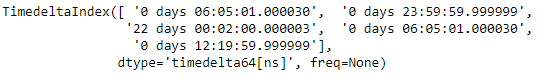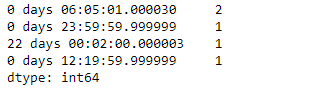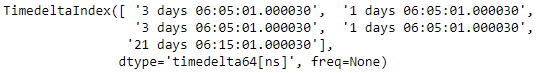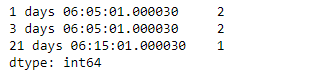Related Articles
Python | Pandas TimedeltaIndex.value_counts()
• Last Updated : 06 Jan, 2019

Python is a great language for doing data analysis, primarily because of the fantastic ecosystem of data-centric python packages. Pandas is one of those packages and makes importing and analyzing data much easier.

Pandas` TimedeltaIndex.value_counts()` function return an object containing counts of unique values. The resulting object will be in descending order so that the first element is the most frequently-occurring element. Excludes NA values by default.

Syntax : TimedeltaIndex.value_counts(normalize=False, sort=True, ascending=False, bins=None, dropna=True)

Parameters :
normalize : (boolean, default False) If True then the object returned will contain the relative frequencies of the unique values.
sort : (boolean, default True) Sort by values
ascending : (boolean, default False) Sort in ascending order
bins: (integer, optional) Rather than count values, group them into half-open bins, a convenience for pd.cut, only works with numeric data
dropna : (boolean, default True) Don’t include counts of NaN.

Return : counts : Series

Example #1: Use `TimedeltaIndex.value_counts()` function to count the occurrence of each unique values in the given TimedeltaIndex object.

 `# importing pandas as pd``import` `pandas as pd`` ` `# Create the TimedeltaIndex object``tidx ``=` `pd.TimedeltaIndex(data ``=``[``'06:05:01.000030'``, ``'+23:59:59.999999'``,``                        ``'22 day 2 min 3us 10ns'``, ``'06:05:01.000030'``,``                        ``'+12:19:59.999999'``])`` ` `# Print the TimedeltaIndex object``print``(tidx)`

Output :Now we will use the `TimedeltaIndex.value_counts()` function to find the count of occurrence of each unique values in the tidx object.

 `# count occurrences``tidx.value_counts()`

Output :As we can see in the output, the `TimedeltaIndex.value_counts()` function has returned an the count of all unique values in the given TimedeltaIndex object.

Example #2: Use `TimedeltaIndex.value_counts()` function to count the occurrence of each unique values in the given TimedeltaIndex object.

 `# importing pandas as pd``import` `pandas as pd`` ` `# Create the TimedeltaIndex object``tidx ``=` `pd.TimedeltaIndex(data ``=``[``'3 days 06:05:01.000030'``, ``'1 days 06:05:01.000030'``,``                        ``'3 days 06:05:01.000030'``, ``'1 days 06:05:01.000030'``,``                        ``'21 days 06:15:01.000030'``])`` ` `# Print the TimedeltaIndex object``print``(tidx)`

Output :Now we will use the `TimedeltaIndex.value_counts()` function to find the count of occurrence of each unique values in the tidx object.

 `# count occurrences``tidx.value_counts()`

Output :As we can see in the output, the `TimedeltaIndex.value_counts()` function has returned an the count of all unique values in the given TimedeltaIndex object.

Attention geek! Strengthen your foundations with the Python Programming Foundation Course and learn the basics.

To begin with, your interview preparations Enhance your Data Structures concepts with the Python DS Course.

My Personal Notes arrow_drop_up